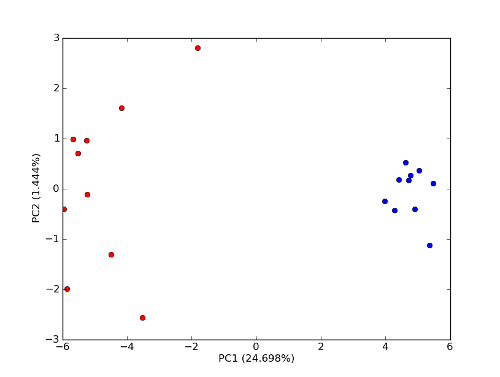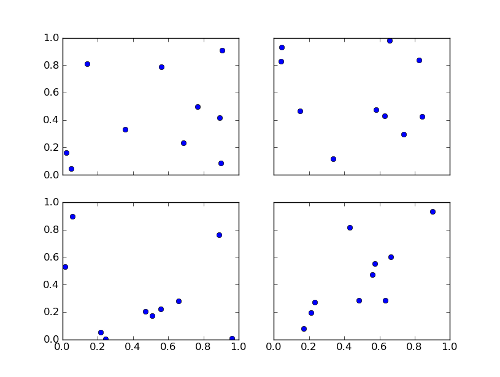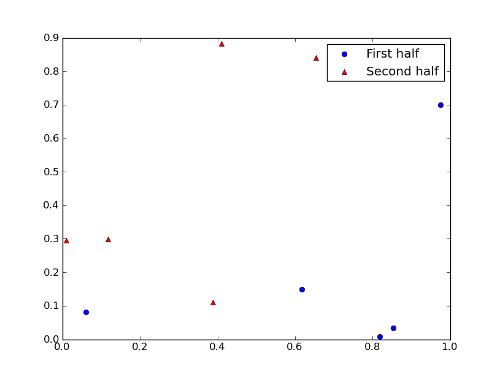## Images and Vision in Python: Slides from talk at Python Edinburgh Mini-Conf 2011

Last weekend the Python Edinburgh users group hosted a mini-conference. Saturday morning was kicked off with a series of talks followed by sessions introducing and then focusing on contributing to django prior to sprints which really got going on the Sunday.

The slides for my talk on, "Images and Vision in Python" are now available in pdf format here.

The slide deck I used is relatively lightweight with my focus being on demonstrating using the different packages available. The code I went through is below.

```from PIL import Image

#Open an image and show it
pil1 = Image.open('filename')
pil1.show()

#Get its size
pil1.size
#Resize
pil1s = pil1.resize((100,100))
#or - thumbnail
pil1.thumbnail((100,100), Image.ANTIALIAS)

#New image
bg = Image.new('RGB', (500,500), '#ffffff')

#Two ways of accessing the pixels

for a in range(100, 200):
for b in range(100,110):
pix[a,b] = (0,0,255)
bg.show()

#Drawing shapes is slightly more involved
from PIL import ImageDraw
draw = ImageDraw.Draw(bg)
draw.ellipse((300,300,320,320), fill='#ff0000')
bg.show()

from PIL import ImageFont
font = ImageFont.truetype("/usr/share/fonts/truetype/freefont/FreeSerif.ttf", 72)
draw.text((10,10), "Hello", font=font, fill='#00ff00')
bg.show()

#Demo's for vision
from scipy import ndimage
import mahotas

#Create a sample image
v1 = np.zeros((10,10), bool)
v1[1:4,1:4] = True
v1[4:7,2:6] = True
imshow(v1, interpolation="Nearest")
imshow(mahotas.dilate(v1), interpolation="Nearest")
imshow(mahotas.erode(v1), interpolation="Nearest")
imshow(mahotas.thin(v1), interpolation="Nearest")

#Opening, closing and top-hat as combinations of dilate and erode

#Labeling
v1[8:,8:] = True
imshow(v1)
labeled, nr_obj = ndimage.label(v1)
nr_obj
imshow(labeled, interpolation="Nearest")
pylab.jet()

#Thresholding
#Convert a grayscale image to a binary image
T = mahotas.otsu(v2)
imshow(v2)
imshow(v2 > T)

#Distance Transforms
dist = mahotas.distance(v2 > T)
imshow(dist)
```

## Quick tips for data analysis in python MDP and matplotlib

I've been using MDP and matplotlib a lot recently and although overall I've been very pleased with the documentation for both projects I have run into a few problems for which the solutions were not immediately obvious. This post gives the solution for each in the expectation it will certainly be useful to me in the future and the hope that it may also be useful to others.

#### Principal Component Analysis with MDP

##### Data Layout

The tutorial for the Modular Toolkit for Data Processing (MDP) starts with a quick example of using the toolkit for a pca analysis and yet I still ran into a couple of problems. The first issue I had was how the pca function expects to receive data. I suspect this is simply due to unfamiliarity with the field and the language used within the field. For future reference the data is expected to be in the following format.

 Gene 1 Gene 2 Gene 3 Gene 4 Experimental Condition 1 . . . . Experimental Condition 2 . . . .
##### Variance Accounted For in PC1, 2, etc

The previously mentioned quick start tutorial was very useful in getting something useful out quickly but I couldn't find a way to get a value for how much of the variance present in the data was accounted for in the principal components. To get that, as far as I've been able to determine, you need to interact with the PCANode directly rather than using the convenience function. The code is still relative straightforward.

```import mdp
import numpy as np
import matplotlib.pyplot as plt

#Create sample data
var1 = np.random.normal(loc=0., scale=0.5, size=(10,5))
var2 = np.random.normal(loc=4., scale=1., size=(10,5))
var = np.concatenate((var1,var2), axis=0)

#Create the PCA node and train it
pcan = mdp.nodes.PCANode(output_dim=3)
pcar = pcan.execute(var)

#Graph the results
fig = plt.figure()
ax.plot(pcar[:10,0], pcar[:10,1], 'bo')
ax.plot(pcar[10:,0], pcar[10:,1], 'ro')

#Show variance accounted for
ax.set_xlabel('PC1 (%.3f%%)' % (pcan.d))
ax.set_ylabel('PC2 (%.3f%%)' % (pcan.d))

plt.show()
```

Running this code produces an image similar to the one below.#### Growing neural gas with MDP

The growing neural gas implementation was another sample application highlighted in the tutorial for MDP. It held my interest for a while as a technique which could potentially be applied to the transcription of plaques for the openplaques project. It wasn't immediately obvious how to get the position of a node from a connected nodes object. As the tutorial left the details of visualisation up to the user I'll present the solution to getting the node location in the form of the necessary code to visualise the node training. The end result will look something like the following.

#### Matplotlib

I've been using Matplotlib to plot data exclusively for a while now. The defaults produce reasonable quality graphs and any differences in opinion can be quickly fixed either by altering options in matplotlib or, as the graphs can be saved in svg format, in a vector image manipulation program such as Inkscape. Although most options can be changed in matplotlib it can sometimes be difficult to find the correct option. Most of the time the naming of variables are, to my mind, logical but sometimes I just can't find the right way to describe what I want to do.

##### Hiding axes

I wanted to have a grid of 6 graphs but didn't want to display the axes on all the graphs as I felt this looked cluttered.

##### Fixing the axis range

If I was going to display the axes on only some of the graphs then the values for the axes needed to be the same on all of them.

```import numpy as np
import matplotlib.pyplot as plt

#Generate sample data
var = np.random.random_sample((40,2))

fig = plt.figure()
for i in range(4):
ax = fig.add_subplot(220 + i + 1)
start = i * 10
ax.plot(var[start:start+10,0], var[start:start+10,1], 'bo')

#Hide the x axis on the top row of charts
if i in [0,1]:
ax.set_xticklabels(ax.get_xticklabels(), visible=False)

#Hide the y axis on the right column of charts
if i in [1,3]:
ax.set_yticklabels(ax.get_yticklabels(), visible=False)

#Set the axis range
ax.axis([0,1,0,1])
plt.show()
```

Running this code should produce an image similar to the one below.##### Removing second point in plot legend

The legend assumes that values are connected so two points and the connecting line are shown by default. If the points on the graph aren't connected then this looked strange. To remove the duplicate symbol is straightforward.

```import numpy as np
import matplotlib.pyplot as plt

#Generate sample data
var = np.random.random_sample((10,2))

#Plot data with labels
fig = plt.figure()